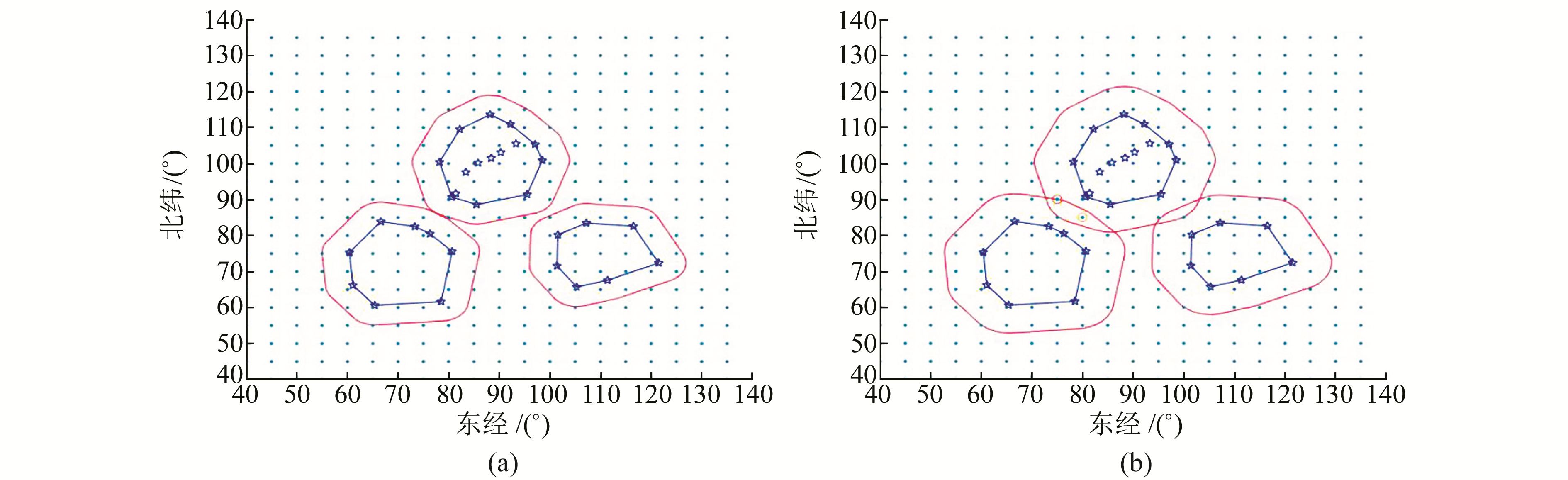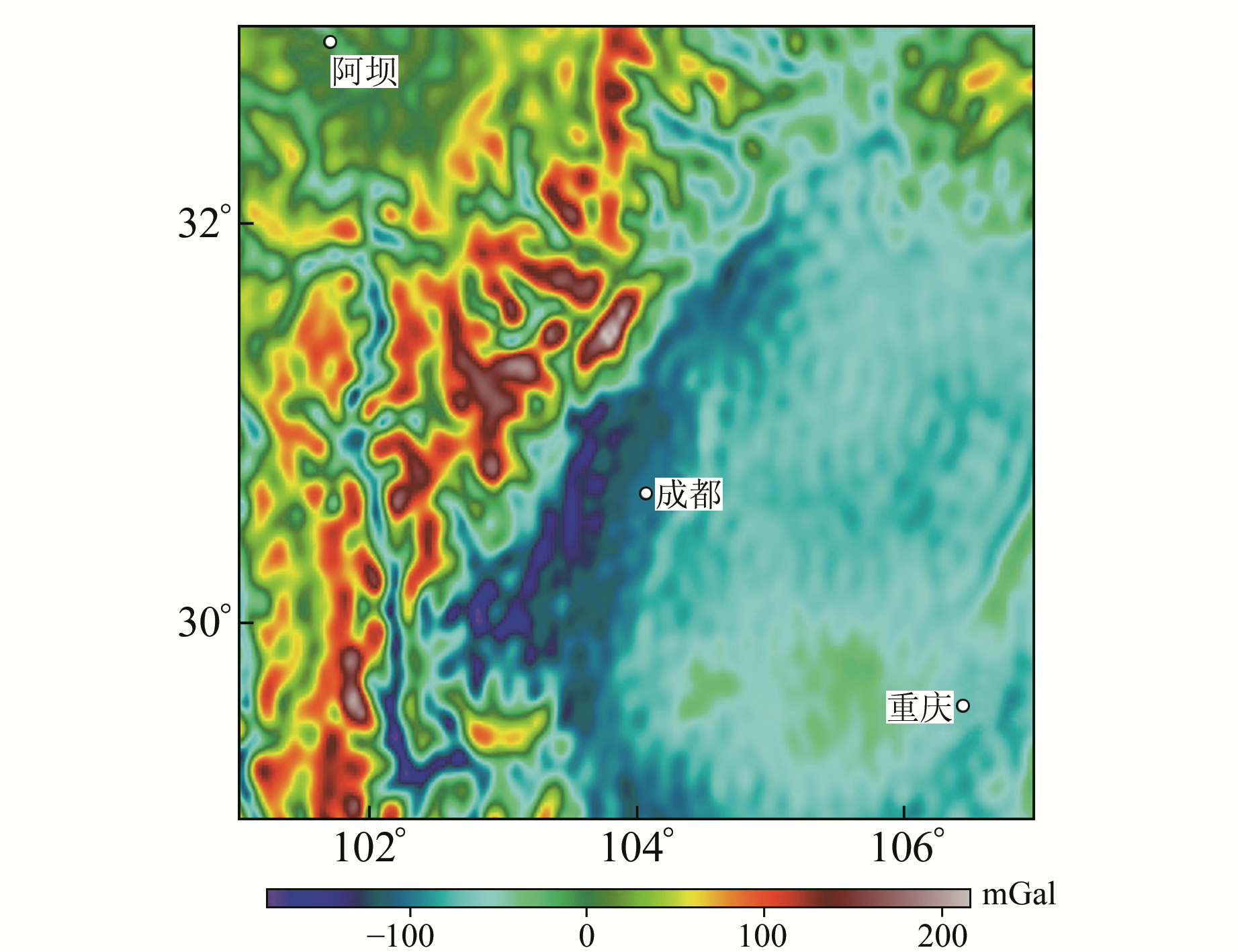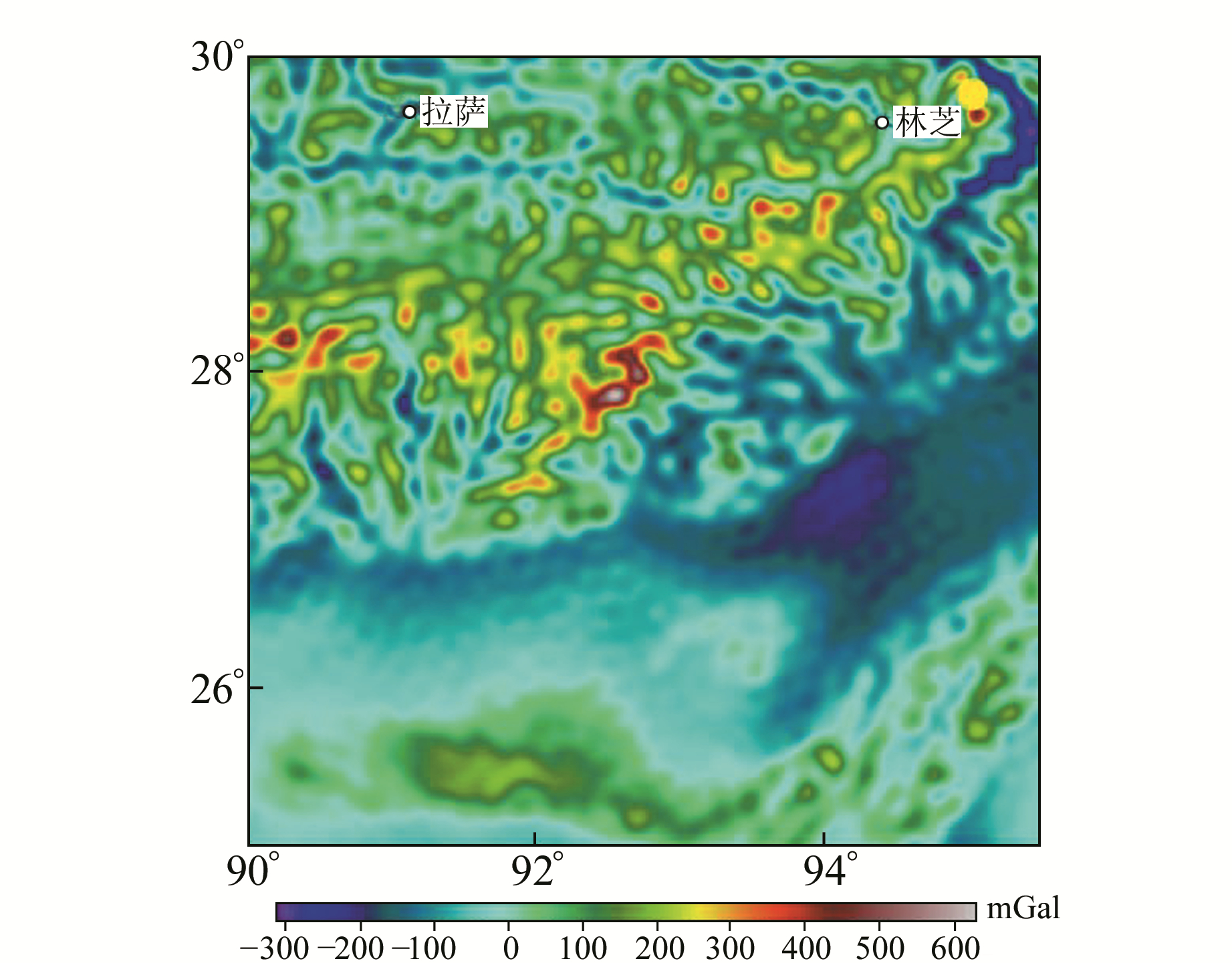﻿ 移去-恢复法校准重力异常场及其应用文章快速检索 高级检索
 大地测量与地球动力学2019, Vol. 39Issue (4): 361-365,431  DOI: 10.14075/j.jgg.2019.04.006### 引用本文ZHAO Bingjie, YANG Guangliang, LI Zhejun. Study on the Remove-Restore Theory of Refining Gravity Anomaly and Its Application[J]. Journal of Geodesy and Geodynamics, 2019, 39(4): 361-365,431.### Foundation support

The Spark Program of Earthquake Technology of CEA, No.XH17022;Scientific Research Fund of Institute of Seismology and Institute of Crustal Dynamics, CEA, No.IS20146141.

### 第一作者简介

ZHAO Bingjie, postgraduate, majors in data processing of geophysics, E-mail:zbj_edu@126.com.

### 文章历史

1. 中国地震局地震研究所地震大地测量重点实验室，武汉市洪山侧路40号，430071;
2. 安徽省地震局，合肥市长江西路558号，230031

1 移去-恢复法算法优化

 $\Delta g_{{\rm{res}}}^r = {g_m}-g_{{\rm{EGM}}}^r$ (1)

 ${g_{{\rm{merge}}}} = \Delta g{\prime _{{\rm{res}}}} + {g_{{\rm{EGM}}}}$ (2)

1.1 插值方法

1) 利用一系列实测点数据的相互位置关系h计算得到一系列变异函数γ(h)，选取球状模型作为变异函数的理论模型进行γ(h)值拟合：

 $\gamma \left( h \right) = \left\{ \begin{array}{l} 0, \;h = 0\\ {c_0} + c\left( {\frac{{3h}}{{2a}}-\frac{{{h^3}}}{{2{a^3}}}} \right), 0 < h \le a\\ {c_0} + c, h > a \end{array} \right.$ (3)

2) 在待测网格点x周围有n个实测点数据，每个实测点的重力异常值为Z(xi), 则克里金插值公式为：

 ${Z^*}\left( x \right) = \sum\limits_{i = 1}^n {{\lambda _i}Z({x_i})}$ (4)

3) 克里金插值的实质是根据测量数据的空间分布特点和变异函数的结构特点对网格点区域化变量取值所进行的线性无偏和最优估计，即

 $\left\{ \begin{array}{l} \sum\limits_{j = 1}^n {{\lambda _j}c} ({x_i}, {x_j})-\mu = c({x_i}, x)\\ \sum\limits_{i = 1}^n {{\lambda _i}} = 1 \end{array} \right.$ (5)

4) 通过式(5)的求解可得克里格估计方差σE2，即

 ${\sigma _E}^2 = c\left( {x, x} \right)-\sum\limits_{i = 1}^n {{\lambda _i}} c({x_i}, x) + \mu$ (6)

5) 在缓冲区外部采用反距离权重插值，分别计算待测网格点坐标与最邻近的一定数量实测点之间的距离，将距离的倒数看作权重，进而将一定数量的实测点重力异常值加权平均得到待测网格点处的重力异常值，即

 ${Z^*}({x_0}) = \frac{{\sum\limits_{i = 1}^n {\frac{1}{{{{({D_i})}^p}}}} Z({x_i})}}{{\sum\limits_{i = 1}^n {\frac{1}{{{{({D_i})}^p}}}} }}$ (7)

1.2 考虑地形梯度

1) 通过归一化对地形梯度变量进行线性变化，将变量值映射到[0, 1]之间，消除变量量纲对结果的影响。变换函数为：

 $x\prime = \frac{{x-{\rm{min}}}}{{{\rm{max-min}}}}$ (8)

2) 再通过标准化处理，使梯度变量满足均值E(x″) = 1，标准差σ(x″) = 1。E(x″) = 1能够将梯度变量当成权重变量，σ(x″) = 1确保不同类型的权重变量对插值结果的最终贡献相同。变换函数为：

 $x" = \frac{{x'-\mu + 1}}{\sigma }$ (9)
1.3 更新重力场区域的划分

1) 根据测网的数量、形状和空间分布进行K-means分类，自动计算最优的分类数，保障各个区域测网的独立性。

2) 计算每个类别中测点的外包络线，并根据测网的精度确定缓冲区距离，计算缓冲区。

3) 根据前文的插值方法，在缓冲区内部利用实测点数据进行克里金插值，在缓冲区外部利用反距离权重法进行插值。

4) 若不同缓冲区存在交集部分，说明该区域在多个缓冲区内部，在对交集区域内的待测网格点插值时，综合利用缓冲区并集中各个实测点进行移去-恢复法插值计算。

 ${\rm{CH}}\left( k \right) = \frac{{{\rm{tr}}\mathit{\boldsymbol{B}}\left( k \right)/\left( {k-1} \right)}}{{{\rm{tr}}\mathit{\boldsymbol{W}}\left( k \right)/\left( {n-k} \right)}}$ (10)图 1 改进移去-恢复法范围分析示意 Fig. 1 Range analysis example diagram
2 川西地区重力场校正分析图 2 川西地区自由空气重力异常图 Fig. 2 Free air gravity anomalous map in west Sichuan表 1 不同方法计算重力场模型标准差 Tab. 1 Different methods for calculating standard deviation of gravity field model

3 喜马拉雅东构造结及邻区重力场校正图 3 喜马拉雅东构造结地形 Fig. 3 Eastern Himalayan syntaxis topographic map图 4 喜马拉雅东构造结自由空气重力异常 Fig. 4 Eastern Himalayan syntaxis free air gravity anomaly map图 5 喜马拉雅东构造结布格重力异常 Fig. 5 Eastern Himalayan syntaxis Bouguer gravity anomaly map图 6 喜马拉雅东构造结均衡重力异常 Fig. 6 Eastern Himalayan syntaxis isostatic gravity anomaly map

4 结语

1) 移去-恢复法能够有效融合多元重力数据，利用少量的实测数据，更新较低精度的重力场，并能有效减弱高频信号的影响。通过对测网的空间位置分布进行分析，自动识别测网间的空间关系，并建立缓冲区，进而在不同区域内分别采用克里金插值和反距离权重插值法，在保证重点插值区域精度的同时，提高了插值效率。

2) 验证了地形梯度因素会对移去-恢复法产生影响的假设。地形梯度变化大，重力异常值通常也会发生较为剧烈的变化，其变化主要是由地表附近的地形起伏引起，而非地球深部物质密度改变造成，故地形梯度变化较大的区域参与待测网格点插值时所赋权重应该较小。利用川西实测数据证明，引入地形梯度变量能够更好地减小重力异常标准差，平滑重力异常场。

3) 通过对喜马拉雅东构造结及邻近地区进行计算分析发现，在喜马拉雅山区南缘地势下降剧烈的区域存在显著的自由空气重力异常高值区，且异常与地形有很好的契合；布格重力异常分布有较强的方向性，整体上呈现南高北低的特点；该区存在3个明显的均衡重力异常区，西部区域地势变化较为剧烈，有2个均衡高值区，而中东部地区存在显著的均衡重力异常低值区。

  宁津生, 罗志才, 杨沾吉, 等. 深圳市1 km高分辨率厘米级高精度大地水准面的确定[J]. 测绘学报, 2003, 32(2): 102-107 (Ning Jinsheng, Luo Zhicai, Yang Zhanji, et al. Determination of Shenzhen Geoid with 1 km Resolution and Centimeter Accuracy[J]. Acta Geodaetica et Cartographica Sinica, 2003, 32(2): 102-107 DOI:10.3321/j.issn:1001-1595.2003.02.002) (0)  荣敏, 周巍, 陈春旺. 重力场模型EGM2008和EGM96在中国地区的比较与评价[J]. 大地测量与地球动力学, 2009, 29(6): 123-125 (Rong Min, Zhou Wei, Chen Chunwang. Evaluation of Gravity Field Models EGM2008 and EGM96 Applied in Chinese Area[J]. Journal of Geodesy and Geodynamics, 2009, 29(6): 123-125) (0)  陆彩萍, 伍吉仓, 王解先. 顾及EGM96模型的GPS水准高程拟合[J]. 测绘工程, 2002, 11(3): 31-34 (Lu Caiping, Wu Jicang, Wang Jiexian. The GPS/Lever Height Fitting Considering of EGM96[J]. Engineering of Surveying and Mapping, 2002, 11(3): 31-34 DOI:10.3969/j.issn.1006-7949.2002.03.009) (0)  管真, 陈剑杰, 尉伯虎, 等. 基于EGM2008的GPS水准拟合在复杂地形中的应用研究[J]. 大地测量与地球动力学, 2012, 32(4): 120-124 (Guan Zhen, Chen Jianjie, Yu Bohu, et al. Application of GPS Leveling Fitting Based on EGM2008 in Complex Terrain[J]. Journal of Geodesy and Geodynamics, 2012, 32(4): 120-124) (0)  付广裕, 祝意青, 高尚华, 等. 川西地区实测自由空气重力异常与EGM2008模型结果的差异[J]. 地球物理学报, 2013, 56(11): 3761-3769 (Fu Guangyu, Zhu Yiqing, Gao Shanghua, et al. Discrepancies between Free-Air Gravity Anomalies from EGM2008 and the Dense Gravity, GPS Observations at West Sichuan Basin[J]. Chinese Journal of Geophysics, 2013, 56(11): 3761-3769 DOI:10.6038/cjg20131117) (0)  佘雅文, 付广裕, 王灼华, 等. 重力与地形数据揭示的巴颜喀拉块体东缘垂向构造应力场[J]. 地球物理学报, 2017, 60(6): 2480-2492 (She Yawen, Fu Guangyu, Wang Zhuohua, et al. Vertical Tectonic Stress in Eastern Margin of Bayan Har Block Revealed by Gravity and Terrain Data[J]. Chinese Journal of Geophysics, 2017, 60(6): 2480-2492) (0)  滕吉文, 王谦身, 王光杰, 等. 喜马拉雅"东构造结"地区的特异重力场与深部地壳结构[J]. 地球物理学报, 2006, 49(4): 1045-1052 (Teng Jiwen, Wang Qianshen, Wang Guangjie, et al. Specific Gravity Field and Deep Crustal Structure of the 'Himalayas East Structural Knot'[J]. Chinese Journal of Geophysics, 2006, 49(4): 1045-1052 DOI:10.3321/j.issn:0001-5733.2006.04.016) (0)
Study on the Remove-Restore Theory of Refining Gravity Anomaly and Its Application
ZHAO Bingjie1YANG Guangliang1     LI Zhejun2
1. Key Laboratory of Earthquake Geodesy, Institute of Seismology, CEA, 40 Hongshance Road, Wuhan 430071, China;
2. Anhui Earthquake Agency, 558 West-Changjiang Road, Hefei 230031, China
Abstract: The classical remove-restore theory is improved. We propose a regional gravity field calibration algorithm considering the gradient feature of terrain using EGM2008 (the satellite gravity field model), surface gravity/GPS joint observation data and ASTER digital terrain model. Based on the layout of the dynamic gravity regional network in China, the calibration range of the gravity field is more reasonable. Compared with the classical application of removal-restoration method in western Sichuan, the new calculation shows that the standard removal-restoration method model can reduce the standard deviation of the gravity field model data in western Sichuan from 65.09 mGal to 61.24 mGal, and combined with the impact of the terrain gradient, it can be further reduced to 59.31 mGal. In this paper, 283 gravimetric data recently published by the International Gravity Bureau are used to calibrate the gravitational field in the eastern Himalayan syntaxis and adjacent areas. Through the Bouguer gravity anomaly map, it is obvious that there is a strong positive correlation between the Bouguer gravity anomaly and the terrain distribution; furthermore, there is a clear area of low value isostatic anomalies in the central and eastern parts of the study area.
Key words: gravity anomaly; EGM2008 model; terrain gradient; remove-restore theory